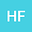Quantum gravity by relativization of Quantum Field Theory.
•• Hontas Farmer
Hontas Farmer
Richard J.Daley College, City Colleges of Chicago

Corresponding Author:hfarmer@ccc.edu

Author Profile## Abstract

The question is how can we make quantum field theory part of General Relativity instead of how we can quantize gravity. Here it will be shown that a Hilbert space can be defined such that the bra-ket is a four vector in ℳ\mathcal{M}inkowski space-time, =va∈ℳ\langle\psi|\phi\rangle=v^{a}\in\mathcal{M}. Similar to a Hilbert space over the field of quarternions. Minkowski space is the tangent space at an arbitrary point on a Riemannian manifold. It will then be shown that the Riemann curvature connects these spaces by operating on the probability density 4-current jaj^{a} of the local QFT. Choosing the Klein-Gordon field as a simple example QFT, the quantized Einstein-Hilbert action will then be derived. From there the expected Feynman diagrams for General Relativity can be read off. In this way one may calculate the gravitational effect due to a quantum field theoretical event.